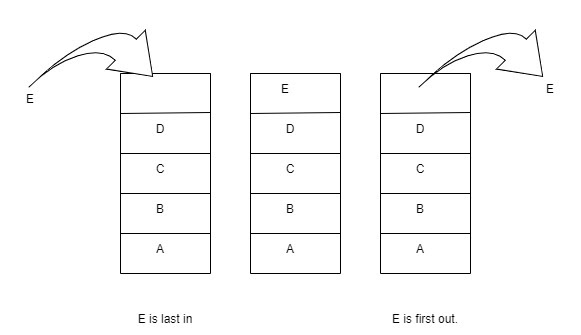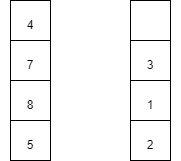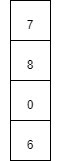# Add two numbers represented by Stacks

## Introduction

This blog will discuss a stack problem frequently asked in Interviews.

The problem is to Add two numbers represented by Stacks.

### Revisiting Stack

Before we proceed to our problem statement, we must know what a Stack is?

A Stack is a linear data structure that stores a list of items, which can be added to or removed from the list only at one end. It is based on LIFO (Last in First Out) mechanism. Stack has four operations: Push, Pop, top, empty.### Problem Statement

Now let’s look at the problem.

We need to add two given numbers when they are represented using stacks. The most significant digit is placed at the bottom of the stack.

For example:

Say, the first number is 5874, and the second number is 213.Output: 6087

## Approach

The approach to Add two numbers represented by Stacks is given below.

Declare two stacks to store both numbers. The most significant digit is placed at the bottom of the stack.

Extract the top element from both the stack and add it.

Push the sum into the stack.

Store the carry and pop out the recently extracted top elements.

Check if the first stack is not empty and move to the new top element. Check the same with the second stack. Calculate the sum and push it in the answer stack. Repeat this process till the stack becomes empty.

If we encounter carry at any step, we need to add it to the sum.

Reverse the answer stack so that the most significant digit is at the bottom of the stack.The figure in LHS represents stack 1 and in RHS is stack 2.

Step 1: top element extracted = 4 and 3.

Their sum=7. Push it into the answer stack.

Step 2: top element extracted = 7 and 1.

Their sum=8. Push 8 into the answer stack.

Step 3: top element extracted = 8 and 2.

Their sum=10. Push 0 into the answer stack. 1 remain as carry

Step 4: Stack 2 becomes empty. 5 is extracted from stack 1.

Their sum=5+1=6. Push 6 into the answer stack. 1 is taken from the carry.

Now the answer array looks like:To get our desired result, we need to reverse our answer stack.

Till now, I guess you must have got the basic idea of what has been asked in the problem statement. So, I strongly recommend you first try it and solve problems related to this.

If you were not able to solve it, don’t be sad.

Please have a look at the algorithm, and then again, you must give it a try.

## PseudoCode

Algorithm

___________________________________________________________________

___________________________________________________________________

1.   stack<int> res

2.   sum = 0, rem = 0

3.   while (!N1.empty() and !N2.empty()):

4.   sum = (rem + N1.top() + N2.top())

5. res.push(sum % 10)

6.    rem = sum / 10

7.    N1.pop()

N2.pop()

8.   while (!N1.empty()):

sum = (rem + N1.top());

res.push(sum % 10);

rem = sum / 10;

N1.pop();

9.   while (!N2.empty()):

sum = (rem + N2.top());

res.push(sum % 10);

rem = sum / 10;

N2.pop();

10. while (rem > 0):

res.push(rem)

rem /= 10

11. while (!res.empty()):

N1.push(res.top());

res.pop();

12. res = N1;

return res;

13. end procedure

___________________________________________________________________

## Implementation in C++

``````// C++ program to Add two numbers represented by Stacks
#include <bits/stdc++.h>

using namespace std;

// Function to calculate the sum of two numbers. Answer will be stored in stack
stack < int > addStack(stack < int > N1, stack < int > N2) {
stack < int > res;
int sum = 0, rem = 0;

while (!N1.empty() and!N2.empty()) {

// Calculate the sum of the top elements of both the stacks
sum = (rem + N1.top() + N2.top());
// Push the sum into the stack
res.push(sum % 10);
// Store the carry
rem = sum / 10;
// Pop the top elements
N1.pop();
N2.pop();
}

// If N1 is not empty
while (!N1.empty()) {
sum = (rem + N1.top());
res.push(sum % 10);
rem = sum / 10;
N1.pop();
}
// If N2 is not empty
while (!N2.empty()) {
sum = (rem + N2.top());
res.push(sum % 10);
rem = sum / 10;
N2.pop();
}

// If carry remains
while (rem > 0) {
res.push(rem);
rem /= 10;
}

// Reverse the stack.so that most significant digit is at the bottom of the stack
while (!res.empty()) {
N1.push(res.top());
res.pop();
}
res = N1;
return res;
}

// Function to display the resultant stack
void display(stack < int > & res) {
int N = res.size();
string s = "";
while (!res.empty()) {
s = to_string(res.top()) + s;
res.pop();
}
cout << s << endl;
}

int main() {
stack < int > N1;
N1.push(5);
N1.push(8);
N1.push(7);
N1.push(4);

stack < int > N2;
N2.push(2);
N2.push(1);
N2.push(3);
stack < int > res = addStack(N1, N2);
display(res);
return 0;
}``````

Output:

``6 0 8 7 ``

#### Complexity Analysis

Time Complexity: O(n)

The above algorithm takes O(n) time complexity, where n is the size of the stack. We traverse the stack only once.

Space complexity: O(n), as we are only introducing an answer stack for storing the answer.

1. What is the difference between stack and array?
Answer) Stack is a dynamic data structure, and it can contain elements of different data types, whereas an array is a static data structure and can hold data of the same type.

2. Why is a stack preferred over an array?
Answer) Due to easy conceptualisation, cleaner code with less logic building.

3. How to understand that a problem can be optimised using a stack?
Answer) If two loops are used, and our j loop depends on i.

## Key Takeaways

This article taught us to Add two numbers represented by Stacks by approaching the problem using the stack approach. We discussed its implementation using illustrations, pseudocode, and then proper code.

We hope you could take away critical techniques like analysing problems by walking over the execution of the examples and finding out the recursive pattern followed in most stack problems.

Now, we recommend you practice problem sets based on stacks to master your fundamentals. You can get a wide range of questions similar to this problem on CodeStudio

It's not the end. Must solve the problem of similar types.

Happy Coding.